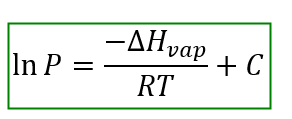# Problem: Suppose the vapor pressure of a substance is measured at two different temperatures.By using the Clausius-Clapeyron equation, large{ln P = -frac {Delta H_{ m vap}}{RT} + C}, derive the relationship between the vapor pressures, P1 and P2, and the absolute temperatures at which they were measured, T1 and T2.

###### FREE Expert Solution

The Clausius-Clapeyron Equation establishes a quantitative relationship between vapor pressure and temperature.Where:

P is the vapor pressure of the species at temperature T

∆Hvap is the enthalpy of vaporization

R is the universal gas constant

T is the temperature in Kelvin

C is an integration constant

100% (223 ratings)###### Problem Details

Suppose the vapor pressure of a substance is measured at two different temperatures.

By using the Clausius-Clapeyron equation, , derive the relationship between the vapor pressures, P1 and P2, and the absolute temperatures at which they were measured, T1 and T2.